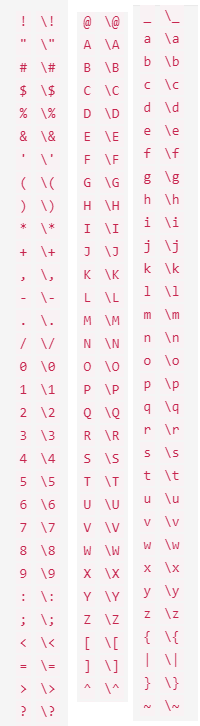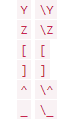# Can I replace character with linefeedchracater in Notepad++ ?

• Using the replace feature in Notepad++, is there a way to replace a character such as: “[” the left bracket say with a combination to effect a “Line-Feed” and then the “[” bracket?

• @Stephen-Knapp

Yes, you would use Regular expression for the Search mode, and specify a Find what box of `[` and then in the Replace with box you would put `\n[`

I swear I was thinking `[` for Find what as I was composing before.

`[` by itself generates `Find: Invalid regular expression` in red at the bottom of the Find window.

• WTF

Did I do it again, or did this site consume my backslash?

I meant `\[`
and so far now I’ve meant it twice!

Edit: Apparently the site ate it, and I did it right the earlier times, I just didn’t proof it.

Normally if you put a single backslash inside backticks, this site will keep it.
But if it is the first character after the opening backtick, it will eat it???

• @Alan-Kilborn
`[` vs `\[`

```````[` vs `\[`
``````

Both look right in preview

Edit: but final render doesn’t match preview

• Now that I’m on a computer, rather than my phone, a fuller experiment:

```````!` `\!`
`"` `\"`
`#` `\#`
`\$` `\\$`
`%` `\%`
`&` `\&`
`'` `\'`
`(` `\(`
`)` `\)`
`*` `\*`
`+` `\+`
`,` `\,`
`-` `\-`
`.` `\.`
`/` `\/`
`0` `\0`
`1` `\1`
`2` `\2`
`3` `\3`
`4` `\4`
`5` `\5`
`6` `\6`
`7` `\7`
`8` `\8`
`9` `\9`
`:` `\:`
`;` `\;`
`<` `\<`
`=` `\=`
`>` `\>`
`?` `\?`
`@` `\@`
`A` `\A`
`B` `\B`
`C` `\C`
`D` `\D`
`E` `\E`
`F` `\F`
`G` `\G`
`H` `\H`
`I` `\I`
`J` `\J`
`K` `\K`
`L` `\L`
`M` `\M`
`N` `\N`
`O` `\O`
`P` `\P`
`Q` `\Q`
`R` `\R`
`S` `\S`
`T` `\T`
`U` `\U`
`V` `\V`
`W` `\W`
`X` `\X`
`Y` `\Y`
`Z` `\Z`
`[` `[`
`]` `]`
`^` `\^`
`_` `\_`
`a` `\a`
`b` `\b`
`c` `\c`
`d` `\d`
`e` `\e`
`f` `\f`
`g` `\g`
`h` `\h`
`i` `\i`
`j` `\j`
`k` `\k`
`l` `\l`
`m` `\m`
`n` `\n`
`o` `\o`
`p` `\p`
`q` `\q`
`r` `\r`
`s` `\s`
`t` `\t`
`u` `\u`
`v` `\v`
`w` `\w`
`x` `\x`
`y` `\y`
`z` `\z`
`{` `\{`
`|` `\|`
`}` `\}`
`~` `\~`
``````

`!` `\!`
`"` `\"`
`#` `\#`
`\$` `\\$`
`%` `\%`
`&` `\&`
`'` `\'`
`(` `\(`
`)` `\)`
`*` `\*`
`+` `\+`
`,` `\,`
`-` `\-`
`.` `\.`
`/` `\/`
`0` `\0`
`1` `\1`
`2` `\2`
`3` `\3`
`4` `\4`
`5` `\5`
`6` `\6`
`7` `\7`
`8` `\8`
`9` `\9`
`:` `\:`
`;` `\;`
`<` `\<`
`=` `\=`
`>` `\>`
`?` `\?`
`@` `\@`
`A` `\A`
`B` `\B`
`C` `\C`
`D` `\D`
`E` `\E`
`F` `\F`
`G` `\G`
`H` `\H`
`I` `\I`
`J` `\J`
`K` `\K`
`L` `\L`
`M` `\M`
`N` `\N`
`O` `\O`
`P` `\P`
`Q` `\Q`
`R` `\R`
`S` `\S`
`T` `\T`
`U` `\U`
`V` `\V`
`W` `\W`
`X` `\X`
`Y` `\Y`
`Z` `\Z`
`[` `[`
`]` `]`
`^` `\^`
`_` `\_`
`a` `\a`
`b` `\b`
`c` `\c`
`d` `\d`
`e` `\e`
`f` `\f`
`g` `\g`
`h` `\h`
`i` `\i`
`j` `\j`
`k` `\k`
`l` `\l`
`m` `\m`
`n` `\n`
`o` `\o`
`p` `\p`
`q` `\q`
`r` `\r`
`s` `\s`
`t` `\t`
`u` `\u`
`v` `\v`
`w` `\w`
`x` `\x`
`y` `\y`
`z` `\z`
`{` `\{`
`|` `\|`
`}` `\}`
`~` `\~`

I skipped the backslash-backslash pair, and the backslash backtick pair, because those both add another layer of confusion. The preview shows all of thse as I would expect:.

Let’s see how it renders after posting…

• Let’s see how it renders after posting…Looks like `\[` and `\]` have special meaning in the forum’s markdown’s backtick implementation. Weird.